# How to Do Long Multiplication

While sitting in an examination hall confronted with a Mathematics exam, all of a sudden you hear the invigilator saying “Ten more minutes to go so kindly check your name and index numbers on the answer sheet”. If you are done with solving this call may not bother you all that much. However, if you have some long calculations and multiplications to make the call seems more like a death sentence. If it is in your control, you would love to glue the invigilator’s lips so that you do not hear anything that may possibly make you lose concentration. Nevertheless, if you know how to do long multiplications in quick time there may be no need to waste the glue for any such purpose.

### Instructions

• 1

First of all you should know about long multiplication as it involves atleast two digits being multiplied with each other. If one number is for instance 4 digits and the other being only one digit then this is not long multiplication.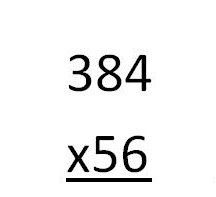• 2

Make sure that in long multiplication you have to multiply each digit of either of the two numbers to be separately in the way shown.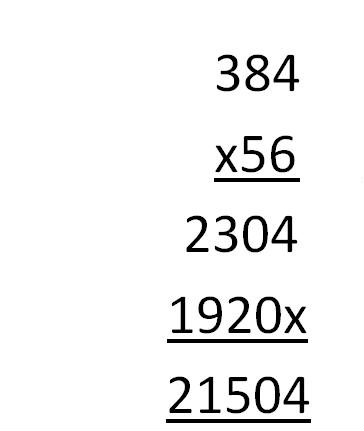• 3

After multiplying the first digit with the number, put a cross or multiplication sign as shown, before multiplying with the other digit with the same number.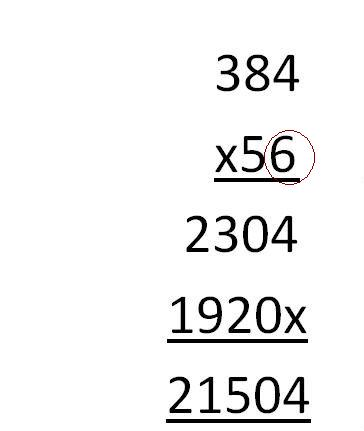• 4

Once you have multiplied both the digits now add both the products that you have obtained separately. The total sum of the both the obtained products will give you the required answer.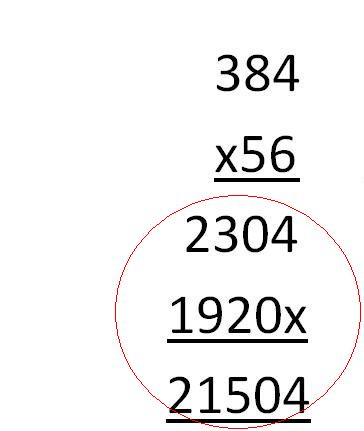• 5

If it seems confusing for you then it is better to first separately multiply each digit with the number and then add the two products as shown.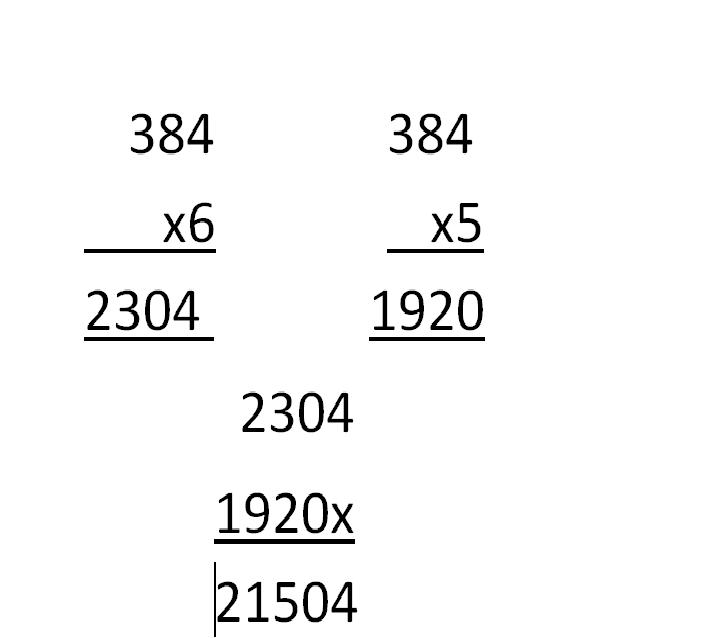•